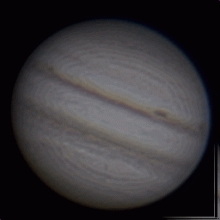# Question about math behind DSLR Intermediate Reduction tutorial

2 posts / 0 new
dario111croQuestion about math behind DSLR Intermediate Reduction tutorial

Because we have three unknowns in the equation, linear algebra requires that we have at least three calibration stars in order to solve for the coefficients, but what happens if one of the calibration stars is mis-measured? We get the wrong coefficients!
It would be nice if we could measure several stars simultaneously and derive the coefficients from that. Fortunately there is a method called least-square fitting that permits us to do so. The mathematics behind this method will be described in the Advanced Reduction tutorial (and in a corresponding JAAVSO article from the DSLR Documentation and Reduction Team), so we will just mention that this method minimizes the chance that a outlying data point can significantly alter the coefficients.

Since advanced reduction tutorial is still not out, I am interested if somebody could explain this shortly. Should I use multiple linear regression?

Regards,
Dario

KBKA
Use simple least-squares fitting

Hi Dario,

I appologize for not noticing this message earlier. I have not been monitoring the Citizen Sky forum on the AAVSO website until I noticed it today.

The DSLR team described the mathematics in this JAAVSO v40n2 pg. 815 (http://www.aavso.org/ejaavso402815). All you need to do is a least-squares fit to the data and propigate the uncertainties as estimated by the least-squares method. The easiest method is to use Linest in Excel (see the documentation for linest on how to get the errors on the coefficients), similar to what was done in the intermediate reduction tutorial.

Cheers,

Brian Kloppenborg

Log in to post comments